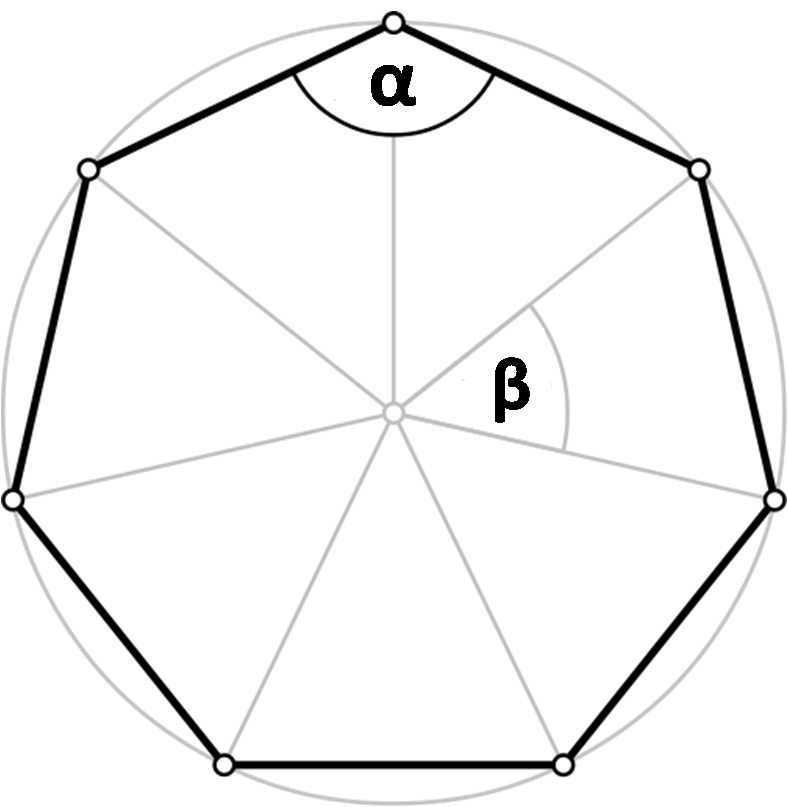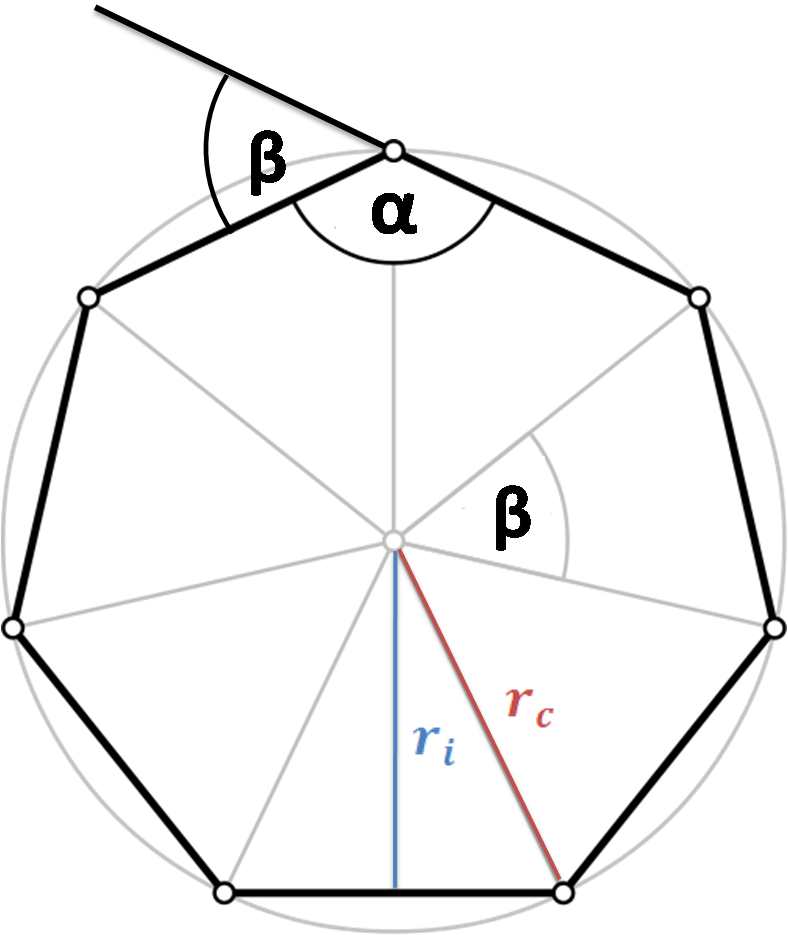# Polygon Calculator

Created by Hanna Pamuła, PhD candidate
Reviewed by Bogna Szyk
Last updated: Nov 09, 2021

With this polygon calculator, you can find the essential properties of any n-sided regular polygon. Whether you are looking for the area of a heptagon or the angles in a decagon, you're at the right place. Below you'll find the polygon definition and a table with the names of polygons along with their shapes. After reading this short article, you'll know what is a polygon and how many sides a particular polygon has - keep reading or simply give this calculator a go!

## What is a polygon? Polygon definition

A polygon is a 2D closed figure made up of straight line segments. That's the polygon definition. But how does it look like? Many shapes you learned about are polygons - triangle, square, parallelogram, rhombus, kite, pentagon, hexagon, octagon... A lot of them. But popular shapes that are not polygons also exist - take a circle and an ellipsis as an example.
Polygons are classified based on their sides and angles, as well as their convexity, symmetry and other properties.In this polygon calculator we solve the regular polygons - the special polygon types which are:

• equiangular - all angles are equal in measure
• equilateral - all sides have the same length

Every regular polygon with n sides is formed by n isosceles triangles.

## How many sides does a polygon have? Names of polygon shapesThe answer to the question depends on which polygon you have on your mind. "Usually, you can use the polygon name as a hint to deduce how many sides it has - the prefixes come from Greek numbers.

Polygon

Name

n (sides)

Regular polygon shape

α

β

3 - sided polygon

trigon (equilateral triangle)

3π/3 = 60°

2π/3 = 120°

4 - sided polygon

4π/2 = 90°

π/2 = 90°

5 - sided polygon

pentagon

53π/5 = 108°

2π/5 = 72°

6 - sided polygon

hexagon

62π/3 = 120°

π/3 = 60°

7 - sided polygon

heptagon (septagon)

75π/7 = 128.57°

2π/7 = 51.43°

8 - sided polygon

octagon

83π/4 = 135°

π/4 = 45°

9 - sided polygon

nonagon

97π/9 = 140°

2π/9 = 40°

10 - sided polygon

decagon

108π/10 = 144°

π/5 = 36°

n - sided polygon

n - gon

n(n-2) * 180°/n

360°/n

If your polygon has 13 or more sides, it's easier to write 13-gon, 14-gon, 20-gon ... "100-gon", etc.

Other polygons

PolygonNamen (sides)αβ
11 - sided polygon Hendecagon (undecagon) 11 147.273° 32.73°
12 - sided polygon Dodecagon 12 150° 30°
13 - sided polygon Triskaidecagon 13 152.308° 27.69°
14 - sided polygon Tetrakaidecagon 14 154.286° 25.71°
15 - sided polygon Pentadecagon 15 156°
24°
16 - sided polygon Hexakaidecagon 16 157.5°
22.5°
17 - sided polygon Heptadecagon 17 158.824°
21.18°
18 - sided polygon Octakaidecagon 18 160°
20°
19 - sided polygon Enneadecagon 19 161.053°
18.98°
20 - sided polygon Icosagon 20 162°
18°
30 - sided polygon Triacontagon 30 168°
12°
40 - sided polygon Tetracontagon 40 171°
50 - sided polygon Pentacontagon 50 172.8°
7.2°
60 - sided polygon Hexacontagon 60 174°
70 - sided polygon Heptacontagon 70 174.857°
5.14°
80 - sided polygon Octacontagon 80 175.5°
4.5°
90 - sided polygon Enneacontagon 90 176°
100 - sided polygon Hectagon 100 176.4°
3.6°
1,000 - sided polygon Chiliagon 1,000 179.64°
0.36°
10,000 - sided polygon Myriagon 10,000 179.964°
0.036°
1,000,000 - sided polygon Megagon 1,000,000 ~180° ~0°
10100 - sided polygon Googolgon 10100 ~180° ~0°

## Regular polygon formulas: sides, area, perimeter, angles

If you want to calculate the regular polygon parameters directly from equations, all you need to know is the polygon shape and its side length:

1. Area
• area = n * a² * cot(π/n)/ 4

Where n- number of sides, a - side length

Other equations, which use parameters such as the circumradius or perimeter, can also be used to determine the area. You can find them in a dedicated calculator of polygon area.

1. Perimeter
• perimeter = n * a

Read more about polygon perimeter in the perimeter of a polygon calculator.1. Angles :
• α = (n - 2) * π / n, where α is an interior angle;
• β = 2 * π / n, where β is an exterior angle.
• ri = a / (2 * tan(π/n))
• rc = a / (2 * sin(π/n))

All these equations are implemented in our polygon calculator.

## How to use this polygon calculator - an example

If you're still wondering how to use our tool, have a look at the following example:

1. Choose the polygon shape and type its number of sides. To calculate the properties of e.g. a nonagon, type 9 into the number of sides box.
2. Enter one parameter. One given value is enough. Assume that we know the perimeter of our shape, let's say it's 18 in.
3. Great! Our polygon calculator finds all the remaining values! We determined that:
• side = 2 in
• area = 24.727 in²
• α = 140°
• β = 40°
• rc = 2.924 in
• ri = 2.7475 in
Hanna Pamuła, PhD candidate
Number of sides
Name
Parameters
Side (a)
in
Perimeter
in
Area
in²
α
deg
β
deg
in
in
People also viewed…

### Improper fraction to mixed number

Use this improper fraction to mixed number calculator to convert quickly between these two fraction forms.

### Lost socks

Socks Loss Index estimates the chance of losing a sock in the laundry.

### Millionaire

This millionaire calculator will help you determine how long it will take for you to reach a 7-figure saving or any financial goal you have. You can use this calculator even if you are just starting to save or even if you already have savings.